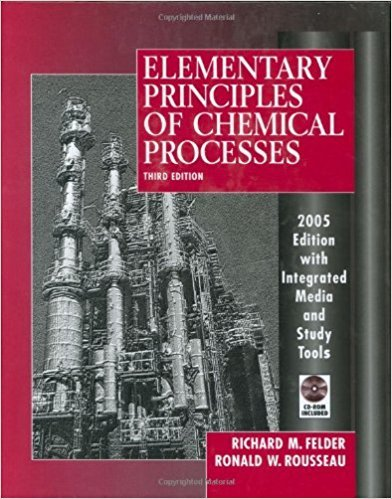×
Get Full Access to Elementary Principles Of Chemical Processes - 3 Edition - Chapter 7 - Problem 7.21
Get Full Access to Elementary Principles Of Chemical Processes - 3 Edition - Chapter 7 - Problem 7.21

×

# The specific enthalpy of liquid n-hexane at 1 atm variesISBN: 9780471687573 143

## Solution for problem 7.21 Chapter 7

Elementary Principles of Chemical Processes | 3rd Edition

• Textbook Solutions
• 2901 Step-by-step solutions solved by professors and subject experts
• Get 24/7 help from StudySoup virtual teaching assistantsElementary Principles of Chemical Processes | 3rd Edition

4 5 1 408 Reviews
13
3
Problem 7.21

The specific enthalpy of liquid n-hexane at 1 atm varies linearly with temperature and equals 25.8 kJ/kg at 30C and 129.8 kJ/kg at 50C. (a) Determine the equation that relates H(kJ/kg) to T(C) and calculate the reference temperature on which the given enthalpies are based. Then derive an equation for U(T)(kJ/kg) at 1 atm. (b) Calculate the average heat transfer rate required to cool 20 kg of liquid n-hexane from 80C to 20C in 5 min.

Step-by-Step Solution:
Step 1 of 3

Step 2 of 3

Step 3 of 3

##### ISBN: 9780471687573

This full solution covers the following key subjects: atm, temperature, liquid, calculate, hexane. This expansive textbook survival guide covers 13 chapters, and 710 solutions. This textbook survival guide was created for the textbook: Elementary Principles of Chemical Processes, edition: 3. Since the solution to 7.21 from 7 chapter was answered, more than 603 students have viewed the full step-by-step answer. The answer to “The specific enthalpy of liquid n-hexane at 1 atm varies linearly with temperature and equals 25.8 kJ/kg at 30C and 129.8 kJ/kg at 50C. (a) Determine the equation that relates H(kJ/kg) to T(C) and calculate the reference temperature on which the given enthalpies are based. Then derive an equation for U(T)(kJ/kg) at 1 atm. (b) Calculate the average heat transfer rate required to cool 20 kg of liquid n-hexane from 80C to 20C in 5 min.” is broken down into a number of easy to follow steps, and 76 words. Elementary Principles of Chemical Processes was written by and is associated to the ISBN: 9780471687573. The full step-by-step solution to problem: 7.21 from chapter: 7 was answered by , our top Chemistry solution expert on 11/15/17, 02:42PM.

Unlock Textbook Solution